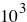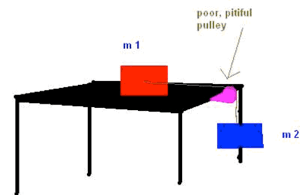# Forces Review

Try these questions and problems to review motion. Mouse over “Answer” to reveal the correct answer.

1) A bag of sugar weighs 5.00 lb on Earth. What should it weigh in N on the moon where the free fall acceleration is 1/6 that on Earth? Repeat for Jupiter where g = 2.64 times that on Earth. Find the mass of the bag of sugar in kg for all 3 locations.

Answer: (3.71 N, 58.7 N, 2.27 kg)

2) A performer in a circus is fired from a cannon as a ‘human cannonball’ and leaves the canon with a speed of 18.0 m/s. The performer’s mass is 80.0 kg. The cannon barrel is 9.20 m long. Find the average net force exerted on the performed while he is being accelerated inside the cannon.

 1.41 XN

)

3) The distance between 2 telephone poles is 50.0 m. When a 1.00 kg bird (big bird!) lands on the telephone wire midway between the poles, the wire sags 0.200 m. Draw a free body diagram of the bird. How much tension does the bird produce in the wire? (Ignore the weight of the wire.)

4) The coefficient of static friction is 0.800 between the soles of a sprinter’s running shoes and the level track surface on which she is running. Determine the maximum acceleration she can achieve. Do you need to know that her mass is 60.0 kg?

 mN=mammg=ma mg=a (0.8)(9.8)=a=7.8m/s2

)

5) An object falling under the pull of gravity is acted upon by a frictional force of air resistance. The magnitude of this force is approximately proportional to the speed of the object, which can be written as f = bv. Assume that b = 14 kg/s and m = 41 kg. What is the terminal speed that the object reaches while falling?

6) A girl coasts down a hill on a sled, reaching a level surface at the bottom with a speed of 7.0 m/s. If the coefficient of friction between the runner on the sled and the snow is 0.050 and the girl and sled together weigh 600 N, how far does the sled travel on the level surface before coming to rest?

7) The coefficient of static friction between the 3.00 kg crate and the 35.0o incline (below) is 0.300. What minimum force F must be applied to the crate perpendicular to the incline to prevent the crate from sliding down the incline?